# MATLAB Live Scripts and Live Editor

## Introduction

The document you are currently reading is a MATLAB Live Script. Live scripts are interactive documents that combine MATLAB code with formatted text, equations, and images in a single interactive environment called the MATLAB Live Editor. In this context you can see your results together with the code that produced them.
In the following sections you will learn
1. How to run, edit and create code with the Live Editor,
2. How to use common operators for basic calculations with numbers,
3. How to define variables to store numbers and perform calculations.

## 1. Getting started: Running code within sections

Each Live Script is divided in sections that may contain code. Each section can be run individually.
To run the code from each section, position the cursor on the code with the mouse and then click on the Run Section button (from the toolstrip) or click on the blue stripe on left side of that section as shown below: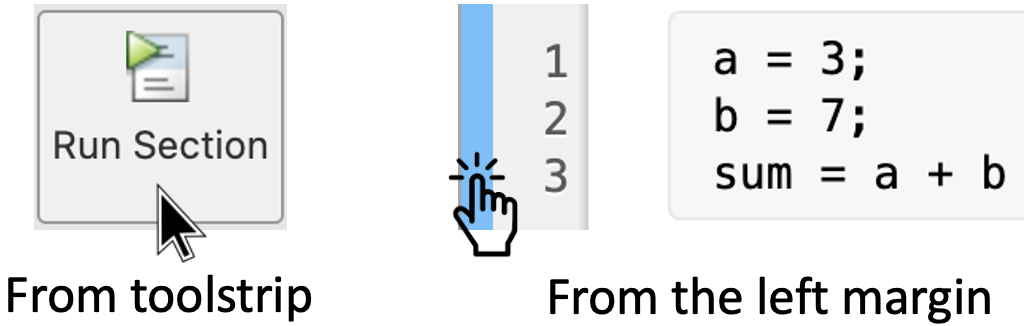Remark: Run the code of each section from top to bottom, otherwise you may get an error.
- The end of a section is indicated with a thin line, like the next one -

### 1.1 MATLAB code and Output display options

In the Live Script the MATLAB code is inside a grey square as the example below which shows the use of the operators +, -, /, * , ^, () for basic calculations with numbers:
2 - 1.3 % Subtraction
8 / 2 % Division
2 * 5 % Multiplication
2^3 % Exponentiation
(3 * 2 - 2) * 2 % Brackets
Note: The text after the percent " % " symbol indicates a comment line in the code.
When you run this section, you will notice that the output appears together with the code inside the live editor. Try it, run this section!
If you click on an output value, the cursor shows you which line of code produced that output as shown image below: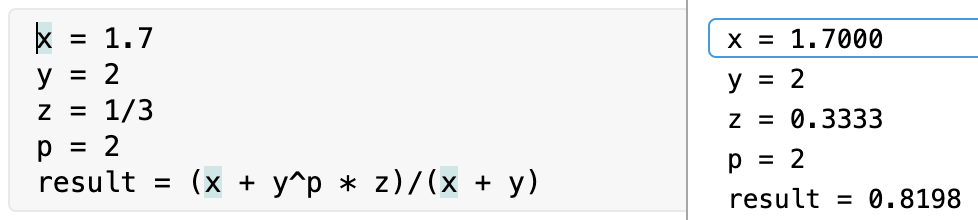You can also display your output inline: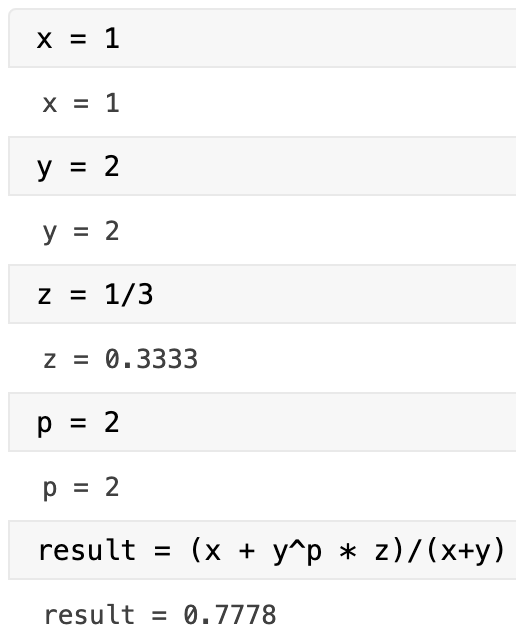To change the output displays, use the icons on the right-side window of the Live Editor: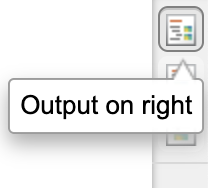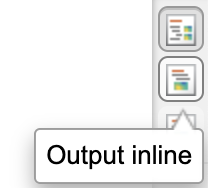### 1.2 Errors

Errors in the code will appear next to the line where they occur: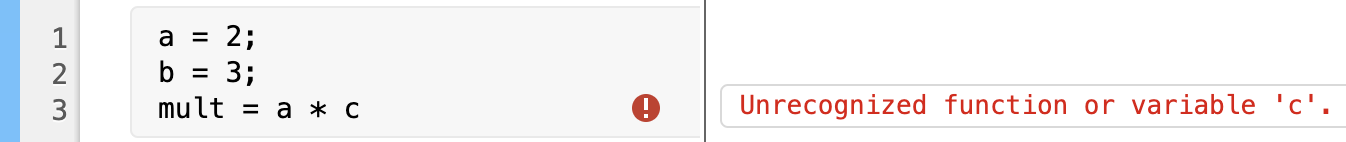The following code has three errors. Run this section to find them. Then fix the errors in the code and re-run it.
a = Pi; % Constant pi
b = Sqrt(2); % Square root of 2
c = Sin(0); % Trig function sin evaluated at 0
op = cos(a / 2) + b + c
Remark: Every time you modify your code a hatch blue bar appears on the left side. This tells you that you have modified the code. To run the latest version just click on this bar.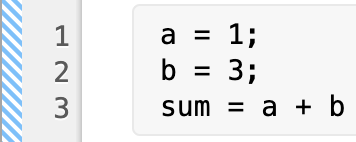### 1.3 Clearing outputs

Finally, if you want to clear all the Outputs, just select any section and click with right button to open the help menu. Then select the option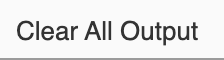## 2. Hands on Practice

Let's practice what we just learned.

### 2.1 Operations with numbers

In section 1.1 we introduced the operators +, -, /, *, ^, () for simple calculations with numbers. In section 1.2 we used the built-in constant pi and the functions sqrt(), sin() and cos().

### Activity 1:

Use these operators and functions to calculate the following:
•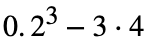•••Write your code here and run it:

### 2.2 Variables to store numbers and calculations

We can define variables to store numbers and operate with them. Variable names consist of a letter followed by any number of letters, digits or underscore. For example:
x = 3;
y_0 = 2
y_1 = 5
initial_value = 0
z0 = x + y_0 * y_1 + initial_value / 2
Remark: Notice that at the beggining of the previous code, the semicolon ";" was added at the end. In general, a semicolon indicates end of statement. However, if you want to suppress and hide the MATLAB output for an expression, you must add a semicolon after the expression. Modify the code to suppress on the output the variables y_0, y_1 and initial_value. Then re-run the code.

### Activity 2: Population growth and carrying capacity

The population growth of an organism can be modelled with the functionwhere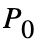represents the initial population (population of the organism at time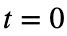), r is the yearly growth rate; and K represents the carrying capacity which is defined to be the maximum population of that organism that the environment can sustain indefinitely.
Problem: A population of rabbits in a meadow is observed to be 200 rabbits at time. Using an initial population of 200 and a yearly growth rate of 0.48, with a carrying capacity of 750 rabbits, calculate the approximate population of rabbits after 2 years.